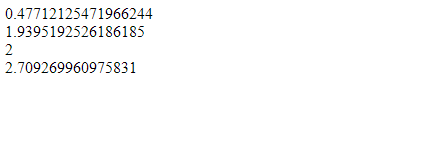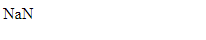# Math.log10() Method with Example in JavaScript

JavaScript | Math.log10() Method: Here, we are going to learn about the log10() method of Math class in JavaScript with Examples.
Submitted by Shivang Yadav, on December 05, 2019

## JavaScript | Math.log10() Method

Math operations in JavaScript are handled using functions of math library in JavaScript. In this tutorial on Math.log10() method, we will learn about the log10() method and its working with examples.

Math.log10() is a function in math library of JavaScript that is used to support math's operation of finding logarithm with base 10 of a number.

Syntax:

```    Math.log10(n);
```

Parameter(s):

• n – It takes only one value which is the number whose logarithm 10 (log10) is to be found.

Return value:

The return type of this method is number, it returns the log10 of the given number.

Example 1: Finding the log10 of number

```<!DOCTYPE html>

<html>

<title>Page Title</title>

<body>
<script>
document.write(Math.log10(3) + "<br>");
document.write(Math.log10(87) + "<br>");
document.write(Math.log10(100) + "<br>");
document.write(Math.log10(512));
</script>
</body>

</html>
```

OutputExample 2: finding log of a string.

```<!DOCTYPE html>

<html>

<title>Page Title</title>

<body>

<script>
document.write(Math.log10("Include help"));
</script>

</body>

</html>
```

OutputWhen a string is passed to the log10() method. It returns NaN denoting the passed value is not a number.

Example 3: Find the log of a complex number.

```<!DOCTYPE html>
<html>

<title>Page Title</title>

<body>

<script>
document.write(Math.log10(1 + 2 i));
</script>

</body>

</html>
```

Output

```Invalid or unexpected token
```

Preparation

What's New

Top Interview Coding Problems/Challenges!